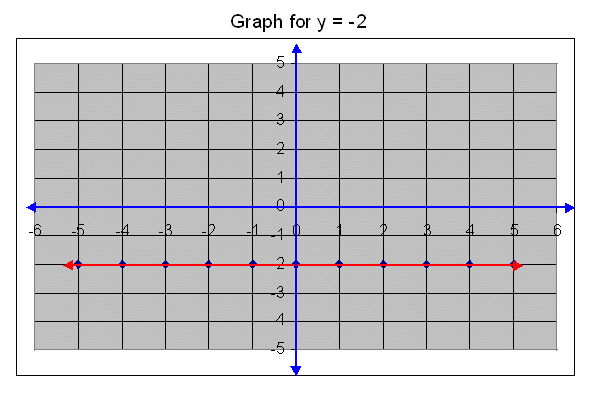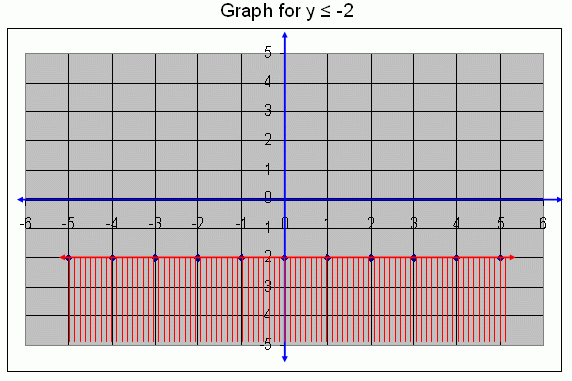Name: ___________________Date:___________________

kwizNET Subscribers, please login to turn off the Ads!
 Email us to get an instant 20% discount on highly effective K-12 Math & English kwizNET Programs!

### Middle/High School Algebra, Geometry, and Statistics (AGS)2.5 Graph for y Ł -2

 Draw a graph for y Ł -2. First we draw a graph for a straight line y = -2, it means that in this straight line the y - coordinate is constant that is -2.y Ł -2, means that y = -2 or y <-2, all these points are satisfies that given condition. Graph for y Ł -2.Directions: Draw the graphs for given conditions> 1. Draw a graph for y Ł -4. 2. Draw a graph for y Ł -1. 3. Draw a graph for y Ł -3. 4. Draw a graph for y Ł -5.Name: ___________________Date:___________________